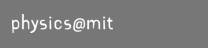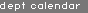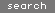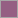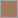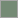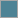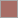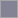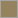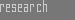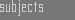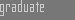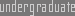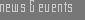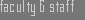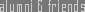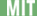> Home > General Info > Experiments > Problem Sets > Quizzes & Exams > FAQs > Staff > Feedback > Grades > Lecture Notes##8.02X :: Electricity & MagnetismQ: Question 4 on the pset 2 was really hard. Will there be questions like this on the quiz?
Dr. Roland says: No, there are no problems like this one on the quiz. If you got problem 4, everything on the quiz should be easy.
Q: When we say that the potential energy U(r) at a point r is related to the potential V(r) by U(r) = V(r)*q, which charge q do we use?
Dr. Roland says: This question shows a bit of confusion on the connection between electric potential and electric potential energy. If we start from some charged object, like a charged sphere, then this object will modify the space around it by creating an electric field. This field is connected to an electric potential, where the potential difference between two points is given by (minus) the line integral of the field between the two points. Both the potential and the field exist independently of any other charged objects q (test charges).
However, the electric potential energy is a property of a test charge that is brought into the field. Let's try an analogy with gravity. Imagine a hill. If you drive a car from the foot of the hill up to the top, the car will have a certain gravitational potential energy relative to its original position. This potential energy is a property of the car, i.e. if you drive a car with a different mass along the same path, it will have a different potential energy. The hill however represents the 'potential', i.e. it is independent of which you car you drive up there (i.e. which test charge you use) and it is also there when there's no car (test charge) around. To be able to talk about electric potential energy, one has to specify which charge is being used (sign and magnitude) and then one can calculate potential energy differences for this particular testcharge.
Q: What about multi-dimensional integrals in quiz 1? Some of us haven't done that in our math classes yet.
Dr. Roland says: The quiz will not be easy, but the difficulty will not be doing integrals. The whole point of Gauss' Law is to use symmmetry to avoid doing complicated integrals. There will be at least one Gauss' Law question.
Q: What style of questions will be on the quiz?
Dr. Roland says: The question will be similar to the ones on the practice quiz and the non-Giancoli questions on the psets.
Q: Can we use both sides of the letter-size formula sheet?
Dr. Roland says: Sure.B